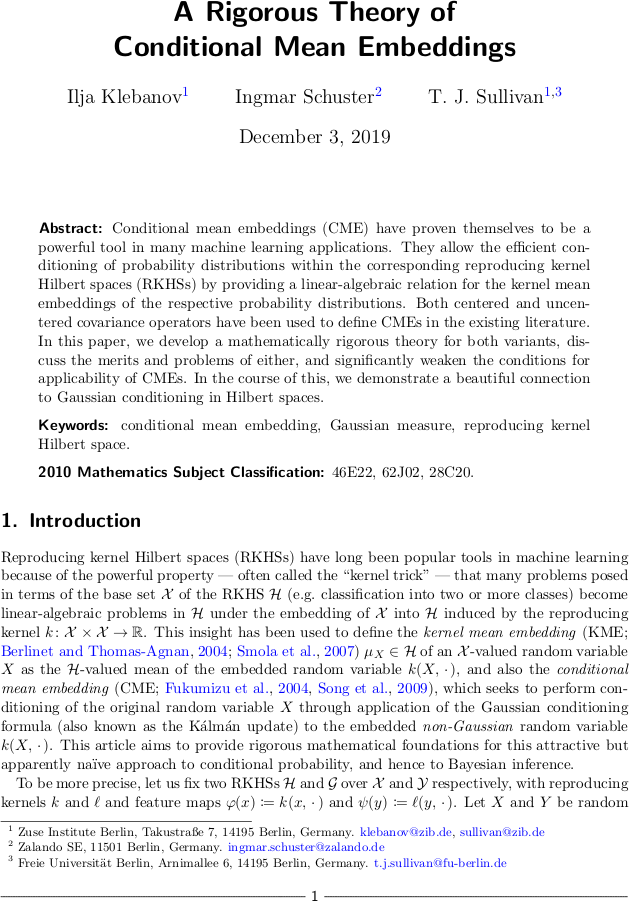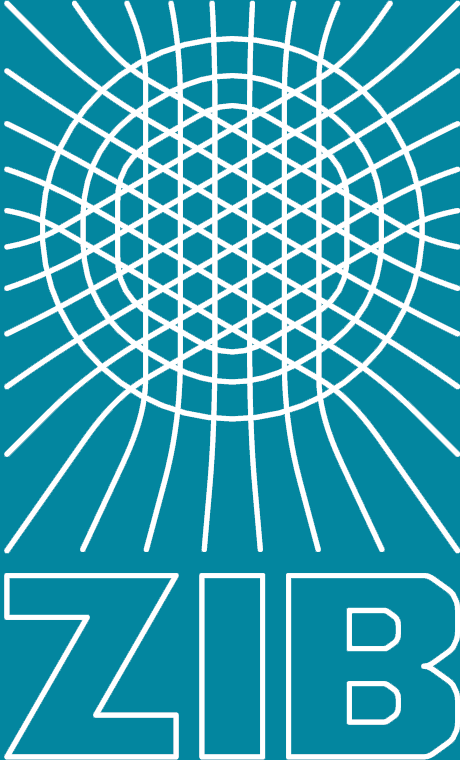# Tim Sullivan

### #mathplus

Clear Search### A rigorous theory of conditional mean embeddings in SIMODS

The article “A rigorous theory of conditional mean embeddings” by Ilja Klebanov, Ingmar Schuster, and myself has just appeared online in the SIAM Journal on Mathematics of Data Science. In this work we take a close mathematical look at the method of conditional mean embedding. In this approach to non-parametric inference, a random variable $$Y \sim \mathbb{P}_{Y}$$ in a set $$\mathcal{Y}$$ is represented by its kernel mean embedding, the reproducing kernel Hilbert space element

$$\displaystyle \mu_{Y} = \int_{\mathcal{Y}} \psi(y) \, \mathrm{d} \mathbb{P}_{Y} (y) \in \mathcal{G},$$

and conditioning with respect to an observation $$x$$ of a related random variable $$X \sim \mathbb{P}_{X}$$ in a set $$\mathcal{X}$$ with RKHS $$\mathcal{H}$$ is performed using the Woodbury formula

$$\displaystyle \mu_{Y|X = x} = \mu_Y + (C_{XX}^{\dagger} C_{XY})^\ast \, (\varphi(x) - \mu_X) .$$

Here $$\psi \colon \mathcal{Y} \to \mathcal{G}$$ and $$\varphi \colon \mathcal{X} \to \mathcal{H}$$ are the canonical feature maps and the $$C$$'s denote the appropriate centred (cross-)covariance operators of the embedded random variables $$\psi(Y)$$ in $$\mathcal{G}$$ and $$\varphi(X)$$ in $$\mathcal{H}$$.

Our article aims to provide rigorous mathematical foundations for this attractive but apparently naïve approach to conditional probability, and hence to Bayesian inference.

I. Klebanov, I. Schuster, and T. J. Sullivan. “A rigorous theory of conditional mean embeddings.” SIAM Journal on Mathematics of Data Science 2(3):583–606, 2020. doi:10.1137/19M1305069

Abstract. Conditional mean embeddings (CMEs) have proven themselves to be a powerful tool in many machine learning applications. They allow the efficient conditioning of probability distributions within the corresponding reproducing kernel Hilbert spaces by providing a linear-algebraic relation for the kernel mean embeddings of the respective joint and conditional probability distributions. Both centered and uncentered covariance operators have been used to define CMEs in the existing literature. In this paper, we develop a mathematically rigorous theory for both variants, discuss the merits and problems of each, and significantly weaken the conditions for applicability of CMEs. In the course of this, we demonstrate a beautiful connection to Gaussian conditioning in Hilbert spaces.

Published on Wednesday 15 July 2020 at 08:00 UTC #publication #simods #mathplus #tru2 #rkhs #mean-embedding #klebanov #schuster### A rigorous theory of conditional mean embeddings

Ilja Klebanov, Ingmar Schuster, and I have just uploaded a preprint of our recent work “A rigorous theory of conditional mean embeddings” to the arXiv. In this work we take a close mathematical look at the method of conditional mean embedding. In this approach to non-parametric inference, a random variable $$Y \sim \mathbb{P}_{Y}$$ in a set $$\mathcal{Y}$$ is represented by its kernel mean embedding, the reproducing kernel Hilbert space element

$$\displaystyle \mu_{Y} = \int_{\mathcal{Y}} \psi(y) \, \mathrm{d} \mathbb{P}_{Y} (y) \in \mathcal{G},$$

and conditioning with respect to an observation $$x$$ of a related random variable $$X \sim \mathbb{P}_{X}$$ in a set $$\mathcal{X}$$ with RKHS $$\mathcal{H}$$ is performed using the Woodbury formula

$$\displaystyle \mu_{Y|X = x} = \mu_Y + (C_{XX}^{\dagger} C_{XY})^\ast \, (\varphi(x) - \mu_X) .$$

Here $$\psi \colon \mathcal{Y} \to \mathcal{G}$$ and $$\varphi \colon \mathcal{X} \to \mathcal{H}$$ are the canonical feature maps and the $$C$$'s denote the appropriate centred (cross-)covariance operators of the embedded random variables $$\psi(Y)$$ in $$\mathcal{G}$$ and $$\varphi(X)$$ in $$\mathcal{H}$$.

Our article aims to provide rigorous mathematical foundations for this attractive but apparently naïve approach to conditional probability, and hence to Bayesian inference.

Abstract. Conditional mean embeddings (CME) have proven themselves to be a powerful tool in many machine learning applications. They allow the efficient conditioning of probability distributions within the corresponding reproducing kernel Hilbert spaces (RKHSs) by providing a linear-algebraic relation for the kernel mean embeddings of the respective probability distributions. Both centered and uncentered covariance operators have been used to define CMEs in the existing literature. In this paper, we develop a mathematically rigorous theory for both variants, discuss the merits and problems of either, and significantly weaken the conditions for applicability of CMEs. In the course of this, we demonstrate a beautiful connection to Gaussian conditioning in Hilbert spaces.

Published on Tuesday 3 December 2019 at 07:00 UTC #preprint #mathplus #tru2 #rkhs #mean-embedding #klebanov #schuster

### Ilja Klebanov joins the UQ Group

It is a pleasure to announce that Ilja Klebanov will join the UQ research group as a postdoctoral researcher with effect from 1 February 2019. He will be working on project TrU-2 “Demand modelling and control for e-commerce using RKHS transfer operator approaches” within the Berlin Mathematics Excellence Cluster MATH+; the project will be led by Stefan Klus and myself.

Published on Friday 1 February 2019 at 09:00 UTC #group #job #tru2 #mathplus #klus #klebanov### Opening for Postdoctoral Researcher

There is an opening in my research group for a postdoctoral researcher in Uncertainty Quantification, for the period 01.01.2019 to 31.12.2020. The position will be associated to the research project TrU-2 “Demand modelling and control for e-commerce using RKHS transfer operator approaches” within the Berlin Mathematics Excellence Cluster MATH+; the project will be led by Stefan Klus and myself. Strong candidates with backgrounds in mathematics, statistics, or computational science are encouraged to apply. For details see the website of the Zuse Institute Berlin, reference number WA57/18. Informal enquiries may be directed to Stefan Klus or to me, and formal applications should be sent to jobs@zib.de by 13.12.2018.

Published on Friday 23 November 2018 at 12:34 UTC #group #job #tru2 #mathplus #klus